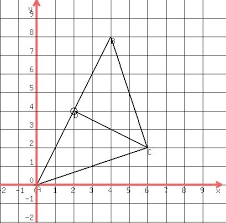# A triangle 10

A triangle has vertices at (4, 5), (-3, 2), and (-2, 5). What are the coordinates of the vertices of the image after the translation (x, y) arrow-right (x + 3, y - 5)?

x0 =  7
y0 =  0
x1 =  0
y1 =  -3
x2 =  1
y2 =  0

### Step-by-step explanation:Did you find an error or inaccuracy? Feel free to write us. Thank you!

Tips for related online calculators